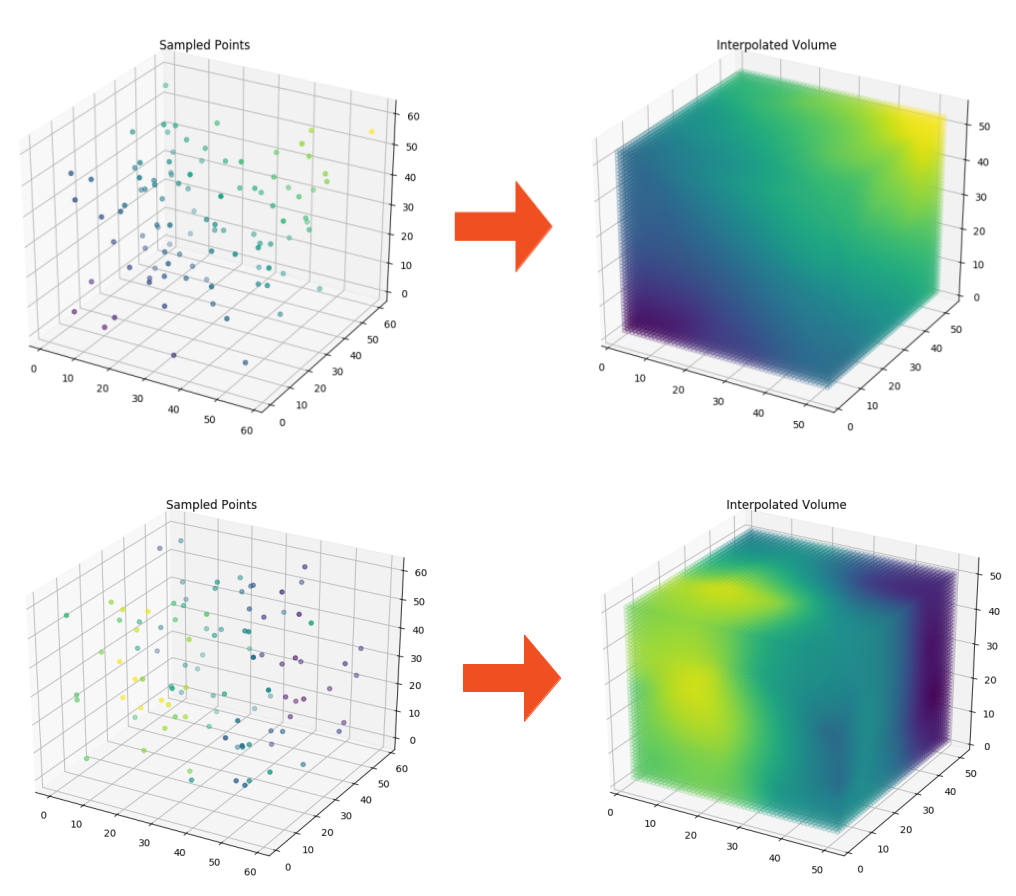# 3D Natural Neighbor Interpolation

For Innolitics

## Project Description

Natural neighbor interpolation is a method for interpolating scattered data (i.e. you know the values of a function at scattered locations). It is often superior to linear barycentric interpolation, which is a commonly used method of interpolation provided by Scipy’s `griddata` function.

There are several implementations of 2D natural neighbor interpolation in Python. We needed a fast 3D implementation that could run without a GPU, so we wrote an implementation of Discrete Sibson Interpolation (a version of natural neighbor interpolation that is fast but introduces slight errors as compared to “geometric” natural neighbor interpolation).

See this paper introducing discrete sibson interpolation for details on the algorithm. You can find the repository for our implementation here.3D Natural Neighbor InterpolationVoroni Diagram